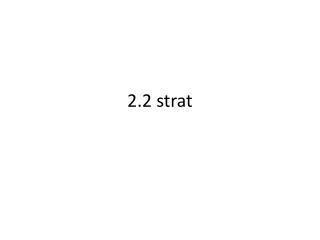DownloadDownload Presentation2.2 strat

# 2.2 strat

Download Presentation## 2.2 strat

- - - - - - - - - - - - - - - - - - - - - - - - - - - E N D - - - - - - - - - - - - - - - - - - - - - - - - - - -
##### Presentation Transcript

1. 2.2 strat

2. Warm UP!!!! find the sum

3. Remember Like terms: Have the same variable and exponent 14x2 12x2 7g 81g 5c 45c 7w3 34w3

4. Match the like terms • 18x • 15y2 • 2m • 7z3 • 9w4 • 17y2 • 3m • 5z4 • 7w4 • 45z3 • 16x

5. You can only add/ subtract like terms!!! 14x + 5x = 19x 12y – 4y = 8y 4c + 5y = NO!!

6. Rules for Exponents: When you add/subtract like terms you keep the exponent the same 3x2 + 5x2 = 8x2 14x2 – 7x2 = 7x2

7. Rules for exponents: When you multiply terms you multiply the coefficients and ADD the exponents. 4x2 ( 8x3 + 5x2) = 32x5 + 20x4 2x( 15x2 + 5x + 4) = 30x3 + 10x2 + 8x

8. Find the product. 2x2(3x2 -5x + 1)

9. Find the product. 3x2( 7x2 +2x +5)

10. You Try!!! Find the product 4x5(3x3 -2x2 – 8x +5)

11. find the product: (w+2)( w2 +8w + 1)

12. Find the product: (5x – 2)( 10x2 –x +5)

13. Find the product: ( 3x + 3) ( 2x – 5)

14. Find the product: ( 6a – 4)( 5a – 1)

15. Simplify the expression: a(3a + 1) + (a + 1)(a + 2)

16. Combined like terms …..

17. You try!! Simplify the expression 2p(p3 +2p) + ( p -3)(p+5)

18. Write a polynomial for the area of the model.

19. Write a polynomial for the area of the model.

20. Classwork: Workbook pg. 67 – 68 # 1 – 20 Homework: Textbook: pg 66 # 1- 20 all

21. Homework: Textbook. Pg. 66 # 1 – 20 all Classwork: Kuta handout